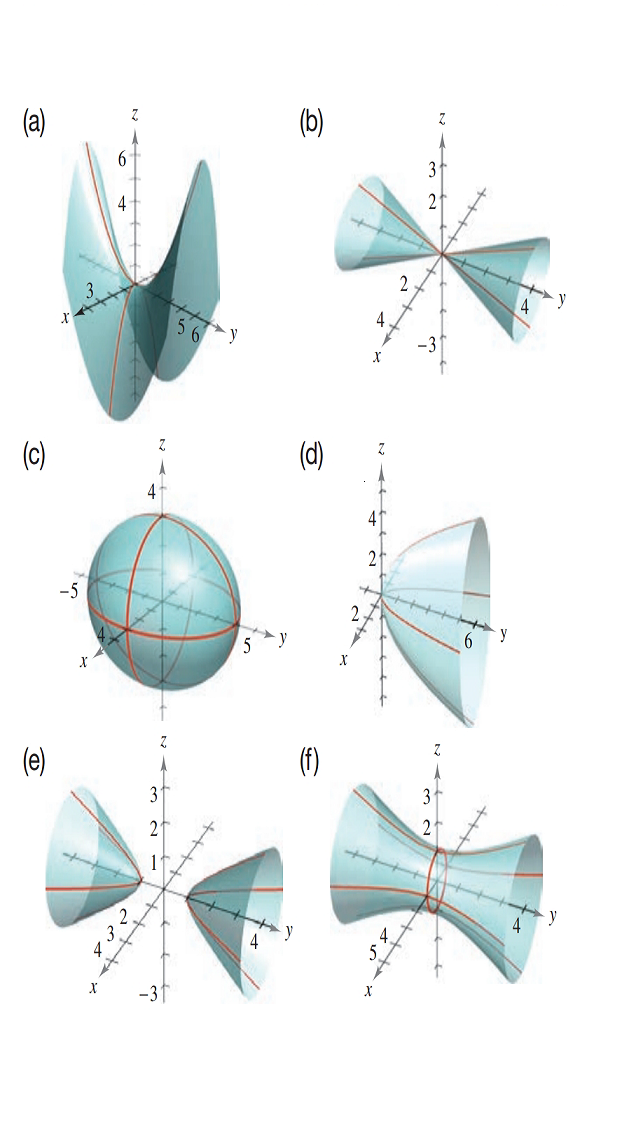Chapter 7.2, Problem 23E### Calculus: An Applied Approach (Min...

10th Edition
Ron Larson
ISBN: 9781305860919

#### Solutions

Chapter
Section### Calculus: An Applied Approach (Min...

10th Edition
Ron Larson
ISBN: 9781305860919
Textbook Problem
1 views

# Matching In Exercises 23-28, match the equation with its graph. [The graphs are labeled (a)-(f).]x 2 9 + y 2 16 + z 2 9 = 1

To determine

The quadratic surface represented by the equation x29+y216+z29=1 and its correct graph from the provided graph.

Explanation

Given Information:

The provided quadratic surface equation is x29+y216+z29=1 and the provided option for matching as follows:

Consider the provided equation x29+y216+z29=1

Rewrite the equation,

x232+y242+z232=1

Since, the standard representation of “hyperboloid of two sheet” in equation form,

x2a

### Still sussing out bartleby?

Check out a sample textbook solution.

See a sample solution

#### The Solution to Your Study Problems

Bartleby provides explanations to thousands of textbook problems written by our experts, many with advanced degrees!

Get Started

#### In Exercises 63-68, use the graph of the function f to determine limxf(x) and limxf(x) 66.

Applied Calculus for the Managerial, Life, and Social Sciences: A Brief Approach

#### For f(x) = tanh1 2x, f(x) = a) 2(sech1 2x)2 b) 2(sech2 2x)1 c) 214x2 d) 114x2

Study Guide for Stewart's Single Variable Calculus: Early Transcendentals, 8th

#### Explain the distinction between a hypothesis and a prediction.

Research Methods for the Behavioral Sciences (MindTap Course List)